# Java Hashmap Example | HashMap in Java

Java HashMap is the part of Collections since JDK1.2. Java HashMap provides the underlying implementation of the Map interface of Java. The HashMap is the hash table based implementation of Java’s Map interface.

A Map, as you might know, is the collection of key-value pairs. It maps keys to values. It stores data in pairs one is key, and another is value.

If we want to access any value, we need a key to it. HashMap uses the Hashing technique that’s why we called it HashMap. In Hashing, we use to convert the long string into a small string, and that represents the same string. A shorter value helps us in indexing and faster searches.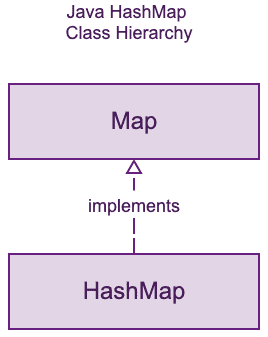## HashMap in Java Example

Arrays store items as the ordered collection and you can access them with an index number which is generally the type of integer. Whereas in HashMap store elements in “key/value” pairs, and you can access them by the key.

One object is used as the key (index) to another object as a value. It can store different types of values such as String keys and Integer values, or the same type, like the String keys and String values.

## Key Points about Java HashMap

The following are some important points about the Java HashMap data structure.

1. It implements the cloneable and serializable interface.
2. HashMap extends an abstract class named AbstractMap, and it provides an implementation of the Map interface.
3. It is a part of java.util package.
4. It doesn’t allow duplicate keys.
5. But it allows duplicate values.
6. It also allows null keys only once and more than one null value.
7. This makes no guarantee as to the order of the map.
8. It can be compared to the Hash Table, but it is synchronized.

## Performance of HashMap

It depends on the following two parameters.

### Initial Capacity

It is the initial size of the number of buckets when a HashMap instance is created. The size of the buckets will be automatically increased if the current size gets full.

It is a measure of how HashMap is allowed to get more buckets before its capacity is automatically increased. When the size gets full of expanding the capacity, the product of the load factor and the current ability, the HashMap is rehashed.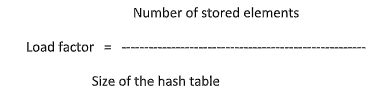## Structure of HashMap

It is nothing but an array of node and a node contains 4 fields:

1. int Hash
2. K key
3. V value
4. Node text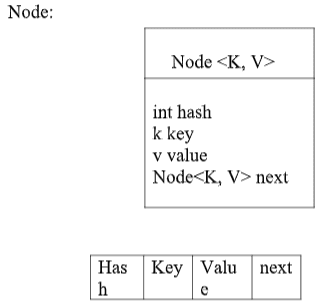Java HashMap class uses the Hash Table to implement the Map interface. It allows the execution time of basic operations, like get() and put(), to remain constant even for large sets.

## Constructor of HashMap

Depending on two parameters there are four types of Constructor are there in HashMap class. We are going to discuss accordingly.

1. HashMap(): It’s a default constructor that has a capacity of 16 and load factor is 0.75.
2. HashMap (int initial capacity): It has initialized initial capacity and specified load factor.
3. HashMap (int initial capacity, float loadFactor): It has both initialized initial capacity and load factor.
4. HashMap (Map map): It creates an instance of HashMap with the same mappings as a specified map.

If we want to use the HashMap class, we need to import the class using the following line of code.

```import java.util.HashMap; // import the HashMap class
```

## How To Create a HashMap

We can create a HashMap using the following code.

```import java.util.HashMap;

public class HashMapC {
public static void main(String[] args) {
HashMap<String, String> shows = new HashMap<String, String>();
}
}```

So, in the above code, we have imported the HashMap class and then created a shows object from the HashMap class. Now, we will add some key/value pair items to that object.

## Add Items in Java HashMap

The HashMap class has many useful methods. For example, to add items to it, use the put() method.

Using the put() method, we can add the key/value pair.

```// HashMapC.java

import java.util.HashMap;

public class HashMapC {
public static void main(String[] args) {

HashMap<String, String> shows = new HashMap<String, String>();
shows.put("Horror", "American Horror Story");
shows.put("Drama", "Game of Thrones");
shows.put("Comedy", "Friends");

System.out.println(shows);
}
}```

The output of the above code is following.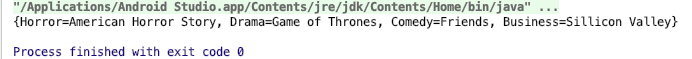## How to Access an Item in HashMap

If we want to access the value of the HashMap then we can use the get() method. See the below code.

```// HashMapC.java

import java.util.HashMap;

public class HashMapC {
public static void main(String[] args) {

HashMap<String, String> shows = new HashMap<String, String>();
shows.put("Horror", "American Horror Story");
shows.put("Drama", "Game of Thrones");
shows.put("Comedy", "Friends");

System.out.println(shows.get("Horror"));
System.out.println(shows.get("Drama"));
System.out.println(shows.get("Comedy"));
}
}```

The output of the above code is following.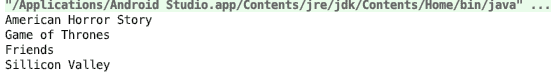## How to Remove an Item in HashMap

If we want to remove any particular item from the HashMap then we can use the key of that HashMap. See the below code.

```// HashMapC.java

import java.util.HashMap;

public class HashMapC {
public static void main(String[] args) {

HashMap<String, String> shows = new HashMap<String, String>();
shows.put("Horror", "American Horror Story");
shows.put("Drama", "Game of Thrones");
shows.put("Comedy", "Friends");

shows.remove("Horror");
shows.remove("Drama");

System.out.println("Remaining items are: " + shows);

}
}
```

See the output.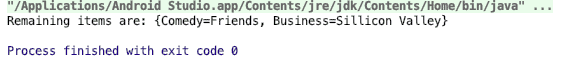If we want to remove all the items then we can use the clear() method.

```// HashMapC.java

import java.util.HashMap;

public class HashMapC {
public static void main(String[] args) {

HashMap<String, String> shows = new HashMap<String, String>();
shows.put("Horror", "American Horror Story");
shows.put("Drama", "Game of Thrones");
shows.put("Comedy", "Friends");

shows.clear();
System.out.println("Remaining items are: " + shows);

}
}```

See the output.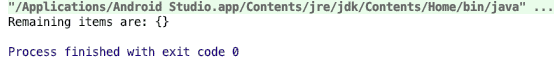## Various Methods in Java HashMap

There are various methods to implement a HashMap in Java. One by one we will discuss them here.

### Object clone() method

It is used to copy the map.

Syntax: hashmap.clone()

It takes no parameters and returns a copy of the mentioned HashMap.

```import java.util.HashMap;

class Hm_clone
{
public static void main(String [] er)
{
//creating an empty hashmap
HashMap<Integer, String> hash_map=new HashMap<>();

//mapping string values to int keys
hash_map.put(10,"AppDividend");
hash_map.put(15,"Welcomes");
hash_map.put(12,"You");

//displaying hash_map
System.out.println("Initial mappings are: "+ hash_map);

//displaying the hashmap after cloning

System.out.println("The cloned map looks like : " + hash_map.clone());
}
}```

See the output.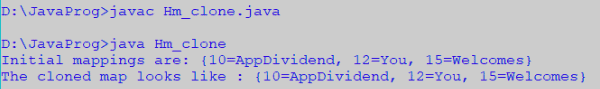### boolean containsKey (Object key)

The containsKey() method is to check if their specified key is present or not.

Syntax: hashmap.containsKey (Object key);

It takes a parameter an object key and returns boolean true if the key is present in HashMap else returns false. See the following code example.

```import java.util.*;

public class Hm_containsKey {
public static void main(String[] args)
{

// Creating an empty HashMap
HashMap<Integer, String> hm = new HashMap<Integer, String>();

// Mapping string values to int keys
hm.put(10, "AppDividend");
hm.put(15, "Welcomes");
hm.put(20, "All");
hm.put(25, "of");
hm.put(30, "You");

// Displaying the HashMap
System.out.println("Initial Mappings are: " + hm);

// Checking for the key_element '20'
System.out.println("Is the key '20' present? " +hm.containsKey(20));

// Checking for the key_element '5'
System.out.println("Is the key '5' present? " +hm.containsKey(5));
}
}```

See the below output.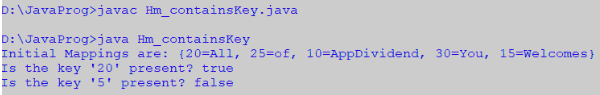### boolean containsValue (Object value)

Here this method is to check whether any particular value is present in HashMap or not.

Syntax: hashmap.containsValue(Object Value);

It takes a specified object value as a parameter and returns true if the passed value is present in the HashMap else it returns false.

See the following code example.

```import java.util.*;

public class Hm_containsValue
{
public static void main(String[] args)
{

// Creating an empty HashMap
HashMap<Integer, String> hm = new HashMap<Integer, String>();

//mapping string values to integer keys
hm.put(17, "AppDividend");
hm.put(15, "Welcomes");
hm.put(20, "All");
hm.put(21, "of");
hm.put(33, "You");

// Displaying the HashMap
System.out.println("Initial Mappings are: " + hm);

// Checking for the Value 'Geeks'
System.out.println("Is the value 'Geeks' present? " +

// Checking for the Value 'World'
System.out.println("Is the value 'World' present? " +
hm.containsValue("World"));
}
}```

See the output.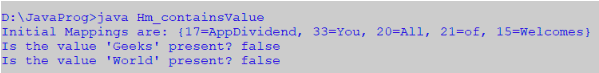### Set entrySet()

It creates a set out of all the same elements in HashMap.

Syntax: hashmap.entrySet();

It takes no parameter but returns a set having the same elements as the HashMap has. See the below code.

```import java.util.*;

public class Hm_entrySet
{
public static void main(String[] args)
{

// Creating an empty HashMap
HashMap<Integer, String> hm = new HashMap<Integer, String>();

// Mapping string values to int keys
hm.put(10, "AppDividend");
hm.put(15, "Welcomes");
hm.put(20, "All");
hm.put(25, "of");
hm.put(30, "You");

// Displaying the HashMap
System.out.println("Initial Mappings are: " + hm);

// Using entrySet() to get the set view
System.out.println("The set is: " + hm.entrySet());
}
}```

See the output.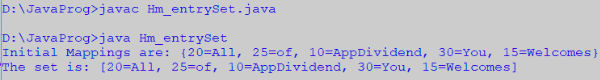### Object get (Object key)

It is used to retrieve the value which is mapped with the specified key value.

Syntax: hashmap(Object key);

It takes a key object as a parameter and returns the value associated with the passed key object.

```import java.util.*;
class Hm_get
{
static public void main(String []er)
{
// Creating an empty HashMap
HashMap<Integer, String> hm = new HashMap<Integer, String>();

// Mapping string values to int keys
hm.put(10, "AppDividend");
hm.put(15, "Welcomes");
hm.put(20, "All");
hm.put(25, "of");
hm.put(30, "You");

// Displaying the HashMap
System.out.println("Initial Mappings are: " + hm);

// Getting the value of 25
System.out.println("The Value is: " + hm.get(25));

// Getting the value of 10
System.out.println("The Value is: " + hm.get(10));
}
}
```

See the output.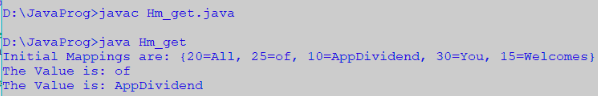### boolean isEmpty()

The isEmpty() method is to check whether the HashMap is empty or not.

Syntax: hashmap.isEmpty();

It takes no parameter but returns boolean true is the HashMap is empty otherwise it returns false.

See the below code.

```import java.util.*;

public class Hm_isEmpty
{
public static void main(String[] args)
{

// Creating an empty HashMap
HashMap<String, Integer> hm = new HashMap<String, Integer>();

// Mapping int values to string keys
hm.put("AppDividend", 10);
hm.put("wlcomes", 15);
hm.put("all", 20);
hm.put("of", 25);
hm.put("You", 30);

// Displaying the HashMap
System.out.println("The Mappings are: " + hm);

// Checking for the emptiness of Map
System.out.println("Is the map empty? " + hm.isEmpty());
}
}```

See the output.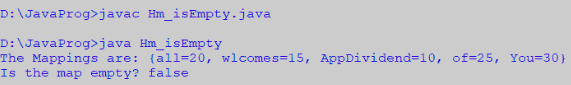### Set keySet()

It is used to create a set of put all the key in the HashMap.

Syntax: hashmap.keySet();

It takes no parameter and returns the set of all the keys contained in HashMap.

See the following code.

```import java.util.*;

public class Hm_keySet
{
public static void main(String[] args)
{

// Creating an empty HashMap
HashMap<Integer, String> hm = new HashMap<Integer, String>();

// Mapping string values to int keys
hm.put(10, "AppDividend");
hm.put(15, "Welcomes");
hm.put(20, "All");
hm.put(25, "of");
hm.put(30, "You");

// Displaying the HashMap
System.out.println("Initial Mappings are: " + hm);

// Using keySet() to get the set view of keys
System.out.println("The set is: " + hm.keySet());
}
}```

See the output.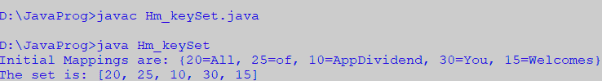### int size()

It helps to get the number of mappings or key-value pairs in HashMap.

Syntax: hashmap.size();

It returns the size of the HashMap.

```import java.util.*;

public class Hm_size
{
public static void main(String[] args)
{

// Creating an empty HashMap
HashMap<Integer, String> hm = new HashMap<Integer, String>();

// Mapping string values to int keys
hm.put(17, "AppDividend");
hm.put(15, "Welcomes");
hm.put(20, "All");
hm.put(21, "of");
hm.put(33, "You");

// Displaying the HashMap
System.out.println("Initial Mappings are: " + hm);

// Displaying the size of the map
System.out.println("The size of the map is " + hm.size());
}
}```

See the output.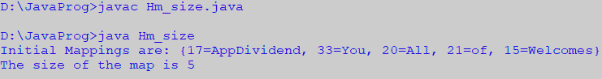### Collection values()

It is used to create a collection of all the values in the HashMap.

Syntax: hashmap.values();

It takes no parameter but returns the set of all the values contained in HashMap. See the below code.

```import java.util.*;

public class Hm_values {
public static void main(String[] args)
{

// Creating an empty HashMap
HashMap<Integer, String> hm = new HashMap<Integer, String>();

// Mapping string values to int keys
hm.put(17, "AppDividend");
hm.put(15, "Welcomes");
hm.put(20, "All");
hm.put(21, "of");
hm.put(33, "You");

// Displaying the HashMap
System.out.println("Initial Mappings are: " + hm);

// Using values() to get the set view of values
System.out.println("The collection is: " + hm.values());
}
}```

See the below output.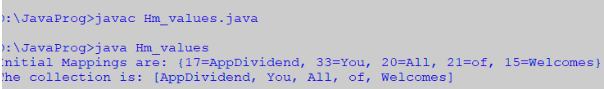## Conclusion

In this article, you have learned how to create a HashMap, how to access the elements of HashMap, how to remove elements and some methods of HashMap.

Conclusively, HashMap in Java Example article is over.

This site uses Akismet to reduce spam. Learn how your comment data is processed.